15 Dec 2021

Posted on:

14 Dec 2021

0

# Resolved:numpy slicing in the for loop

I am in doubt about the slice used in this lesson, i know it is not the core of this excercise. i do not understand this [0, :] in the for loop.

What is the function of the zero + comma before the colon?

Instructor
Posted on:

15 Dec 2021

0

Hey Carlos,

What this notation allows is to perform slicing of N-dimensional `numpy` arrays. Take a look at the example below. I have defined a `numpy` array with three rows (indices 0, 1 and 2) and four columns (indices 0, 1, 2 and 3).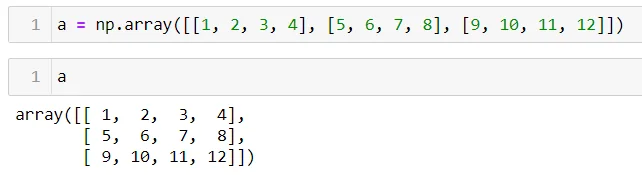Let's do the following: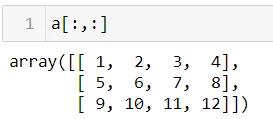The first colon within the brackets reads 'take all rows from the matrix', whereas the second colon reads 'take all columns from the matrix'. Therefore, the comma between the colons separates rows from columns in a matrix. Alright, now, typing `a[0,:]` would slice the matrix such that we take only the row with index 0 together with all the columns. This would produce the following result: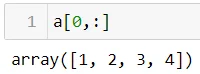Analogously, typing `a[:,0]` would take the numbers from all rows and only the first column: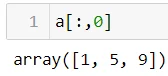You can of course slice the matrix in many different ways. One way which you are probably familiar with is to slice only one number from the matrix. For example `a[0,3]` would output the number in the first row and the fourth column: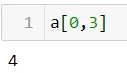I hope this answers your question! Play around with the notation yourself to get a good feeling about what is going on! If you are still in doubt, you can always refer to our 'Data Preprocessing with NumPy' course, Section 4: 'Working with Arrays', Lecture 1: 'Basic Slicing'. There, you can find a very comprehensive explanation on the matter :)

Kind regards,
365 Hristina